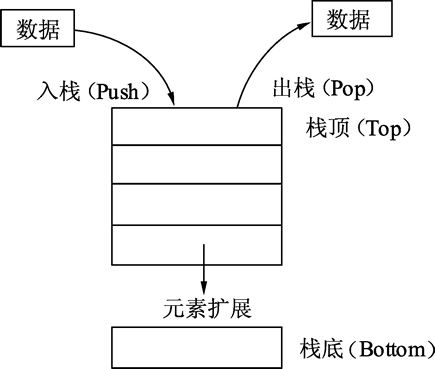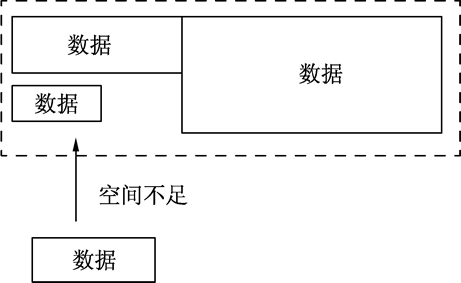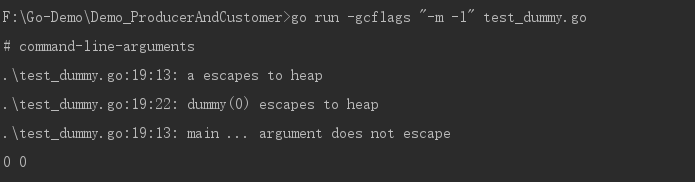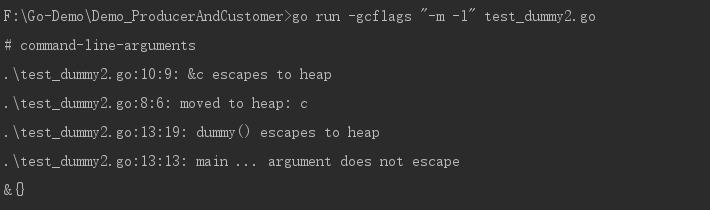15 篇文章 0 订阅

# 1、变量的声明

## 1.1、标准格式

Go语言的变量声明的标准格式为：

var 变量名 变量类型


Go语言的变量类型有：

• bool
• string
• int、int8、int16、int32、int64
• uint、uint8、uint16、uint32、uint64、uintptr
• byte // uint8 的别名
• rune // int32 的别名 代表一个 Unicode 码
• float32、float64
• complex64、complex128

所有的内存在 Go 中都是经过初始化的。

var a, b *int


## 1.2、简短格式

名字 := 表达式


• 定义变量，同时显式初始化。
• 不能提供数据类型。
• 只能用在函数内部。

c,d := 1, "abc"


## 1.3、批量格式

var (
a int
b []float32
c func() bool
d struct {
e int
}
)


# 2、变量的初始化

## 2.1、标准格式

var 变量名 类型 = 表达式


• 整型和浮点型变量的默认值为 0 和 0.0。
• 字符串变量的默认值为空字符串。
• 布尔型变量默认为 bool。
• 切片、函数、指针变量的默认为 nil。

## 2.2、编译器推导类型的格式

var hp = 100


## 2.3、声明并初始化

注意：由于使用了:=，而不是赋值的=，因此推导声明写法的左值变量必须是没有定义过的变量。



var 的变量声明还有一种更为精简的写法，例如：

hp := 100


// 声明 hp 变量
var hp int
// 再次声明并赋值
hp := 10


conn, err := net.Dial("tcp", "127.0.0.1:8080")
conn2, err := net.Dial("tcp", "127.0.0.1:8080")


# 3、多个变量同时赋值

var a int = 100
var b int = 200
b, a = a, b
fmt.Println(a, b)


type IntSlice []int
func (p IntSlice) Len() int           { return len(p) }
func (p IntSlice) Less(i, j int) bool { return p[i] < p[j] }
func (p IntSlice) Swap(i, j int)      { p[i], p[j] = p[j], p[i] }


# 4、匿名变量（没有名字的变量）

匿名变量不占用内存空间，不会分配内存。匿名变量与匿名变量之间也不会因为多次声明而无法使用。

func GetData() (int, int) {
return 100, 200
}
func main(){
a, _ := GetData()
_, b := GetData()
fmt.Println(a, b)
}


100 200


# 5、变量的作用域（即生命周期）

• 函数内定义的变量称为局部变量
• 函数外定义的变量称为全局变量
• 函数定义中的变量称为形式参数

• 全局变量：它的生命周期和整个程序的运行周期是一致的；
• 局部变量：它的生命周期则是动态的，从创建这个变量的声明语句开始，到这个变量不再被引用为止；
• 形式参数和函数返回值：它们都属于局部变量，在函数被调用的时候创建，函数调用结束后被销毁。

## 5.1、局部变量

package main
import (
"fmt"
)
func main() {
//声明局部变量 a 和 b 并赋值
var a int = 3
var b int = 4
//声明局部变量 c 并计算 a 和 b 的和
c := a + b
fmt.Printf("a = %d, b = %d, c = %d\n", a, b, c)
}


## 5.2、全局变量

Go语言程序中全局变量与局部变量名称可以相同，但是函数体内的局部变量会被优先考虑。例如：

package main
import "fmt"
//声明全局变量
var a float32 = 3.14
func main() {
//声明局部变量
var a int = 3
fmt.Printf("a = %d\n", a)
}


a = 3


## 5.3、形式参数

func sum(a, b int) int {
fmt.Printf("sum() 函数中 a = %d\n", a)
fmt.Printf("sum() 函数中 b = %d\n", b)
num := a + b
return num
}


# 6、变量逃逸分析

## 6.1、什么是栈

### 6.1.1、概念### 6.1.2、变量和栈有什么关系

func calc(a, b int) int {
var c int
c = a * b
var x int
x = c * 10
return x
}


## 6.2、什么是堆## 6.3、堆和栈的区别

• 堆（heap）：堆是用于存放进程执行中被动态分配的内存段。它的大小并不固定，可动态扩张或缩减。当进程调用 malloc 等函数分配内存时，新分配的内存就被动态加入到堆上（堆被扩张）。当利用 free 等函数释放内存时，被释放的内存从堆中被剔除（堆被缩减）；
• 栈(stack)：栈又称堆栈， 用来存放程序暂时创建的局部变量，也就是我们函数的大括号{ }中定义的局部变量。

var global *int
func f() {
var x int
x = 1
global = &x
}
func g() {
y := new(int)
*y = 1
}


## 6.4、变量逃逸（Escape Analysis）——自动决定变量分配方式，提高运行效率

Go语言将这个过程整合到了编译器中，命名为“变量逃逸分析”。通过编译器分析代码的特征和代码的生命周期，决定应该使用堆还是栈来进行内存分配。

### 6.4.1、逃逸分析

package main
import "fmt"
// 本函数测试入口参数和返回值情况
func dummy(b int) int {
// 声明一个变量c并赋值
var c int
c = b
return c
}
// 空函数, 什么也不做
func void() {
}
func main() {
// 声明a变量并打印
var a int
// 调用void()函数
void()
// 打印a变量的值和dummy()函数返回
fmt.Println(a, dummy(0))
}


go run -gcflags "-m -l" 文件名.go• 第 2 行告知“代码的第 19 行的变量 a 逃逸到堆”。
• 第 3 行告知“dummy(0) 调用逃逸到堆”。由于 dummy() 函数会返回一个整型值，这个值被 fmt.Println 使用后还是会在 main() 函数中继续存在。
• 第 4 行，这句提示是默认的，可以忽略。

### 6.4.2、取地址发生逃逸

package main
import "fmt"
// 声明空结构体测试结构体逃逸情况
type Data struct {
}
func dummy() *Data {
// 实例化c为Data类型
var c Data
//返回函数局部变量地址
return &c
}
func main() {
fmt.Println(dummy())
}Go语言最终选择将 c 的 Data 结构分配在堆上。然后由垃圾回收器去回收 c 的内存。

### 6.4.3、原则

• 变量是否被取地址；
• 变量是否发生逃逸。点击重新获取扫码支付余额充值